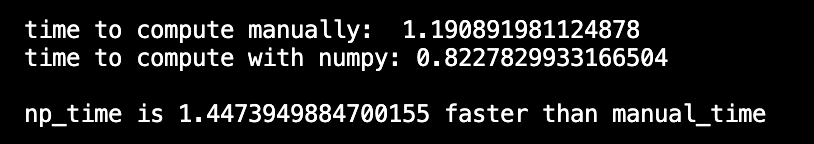All posts

# Programming Libraries & Efficiency: NumPy, Deepgram, PyTorch, and More##### Share

When starting to code with AI, one common question that experienced engineers and data scientists ask is “Why should I use libraries like NumPy to carry out simple calculations that I can code myself?”

But alright, let’s entertain the question for a bit: Yes, it’s a burden to learn a new framework or library or API. Yes, reading documentation can be a chore. Yes, it’s more appealing to code directly from brain to keyboard without having to pause to check syntax.

But trust us, that learning curve is worth the climb.

Let’s use NumPy as a toy example. Pretend that you have a quick, math-y task to take care of: Given two equally long lists of numbers, return an element-wise sum of the lists.

That is, if we’re given the lists `[10, 10, 10]` and `[3, 10, 8]`, then we should return the list `[13, 20, 18]`

Alright, sounds simple enough. Experienced coders like you and me could pull out a cutesy-wootsy for-loop and write some code like this:

``````# Manually compute element-wise sum, given lists `a` and `b`

result = []

for index in range(LENGTH):
sum = a[index] + b[index]
result.append(sum)
return result``````

Great! Why would we ever learn the brand new syntax of a brand new library to do something so simple?

Well, as we mentioned before, the answer is efficiency, but also—in this case—elegance. If we were to use NumPy to accomplish the same task, our code would look as follows:

``````import numpy as np

# Manually compute element-wise sum, given lists `a` and `b`

Fewer lines of code. Faster to read. Faster to run.

No, seriously. It’s faster to run.

NumPy has written their implementations to allow for maximum parallelization, running on machines that are optimized solely for linear algebra. The result is faster outputs that your manually-written code simply cannot compete against.

We put it to the test below. Given two lists of 10 million random integers between -10,000 and 10,000, we computed the element-wise sum manually and with NumPy. Here’s the code:

``````import numpy as np
import random as r
import time as t

LENGTH = 10000000

def main():
a = [r.randint(-10000, 10000) for i in range(LENGTH)]
b = [r.randint(-10000, 10000) for j in range(LENGTH)]

result = []

# Manually compute vector sum
start_manual = t.time()
for index in range(LENGTH):
sum = a[index] + b[index]
result.append(sum)
end_manual = t.time()

# Convert lists into np arrays
a_np = np.array(a)
b_np = np.array(b)

start_np = t.time()
end_np = t.time()

manual_time = end_manual - start_manual
np_time = end_np - start_np

print("time to compute manually: ", str(manual_time))
print("time to compute with numpy:", str(np_time))
print()
print("np_time is " + str(manual_time / np_time) + " faster than manual_time")

main()``````

The result? The NumPy implementation was 1.44x faster than its manual counterpart. Though these exact numbers will differ on your personal machine, the punchline remains: Using the library is much, much faster.

And when the volume of data to process increases from a single list of 10-million numbers to lists of lists of lists of 100 billion numbers each, a 1.44x speed-up becomes crucial—especially in a competitive market.This philosophy of using the most high-level library possible to code up your next app or webpage extends beyond mere math. This extends to AI in general. As a result...

If you’re evaluating a large amount of linear algebra, don’t code from scratch. Use NumPy.

But if you’re coding up a neural network, don’t use NumPy to build one from scratch. Use PyTorch.

And if you’re creating an automated speech-recognition model, don’t use PyTorch to build one from scratch. Use Deepgram.

If you have any feedback about this post, or anything else around Deepgram, we'd love to hear from you. Please let us know in our GitHub discussions .